Do you like the article?
Share it with others -
Use new possibilities of MetaTrader 5

# Probability theory and mathematical statistics with examples (part I): Fundamentals and elementary theory

30 October 2020, 09:08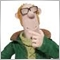0
9 818

### Contents

1. Introduction
2. Theoretical foundations
3. Elementary theory
• 3.1. Combinatorial probability
• 3.2. Bernoulli scheme
4. Fundamentals of mathematical statistics
5. Examples of applying mathematical statistics within the framework of the elementary theory
• 5.1. Parameter point estimation
• 5.2. Testing statistical hypotheses
6. Conclusion
7. Attached files

### 1.Introduction

Trading is always about making decisions in the face of uncertainty. This means that the results of the decisions are not quite obvious at the time these decisions are made. This entails the importance of theoretical approaches to the construction of mathematical models allowing us to describe such cases in meaningful manner. I would like to highlight two such approaches: probability theory and game theory. Sometimes, they are combined together as the theory of "games against nature" in topics related to probabilistic methods. This clearly showcases the existence of two different types of uncertainty. The first one (probabilistic) is usually associated with natural phenomena. The second one (purely game-related) is associated with the activities of other subjects (individuals or communities). The game uncertainty is much more difficult to deal with theoretically. Sometimes, these uncertainties are even called "bad" and "good". The progress in understanding of initially game-related uncertainty is often associated with reducing it to a probabilistic form.

In case of financial markets, the uncertainty of the game nature is obviously more important, since the activity of people is the key factor here. The transition to probabilistic models here is usually based on considerations of a large number of players, each of whom individually has little effect on price changes. In part, this is similar to the approach used in statistical physics, which led to the emergence of a scientific approach called econophysics.

In fact, the topic of such a transition is very interesting, non-trivial, and deserves more detailed consideration. Hopefully, the related articles will appear on our forum someday. In this article, we will look at the very foundations of probability theory and mathematical statistics.

### 2. Theoretical foundations

The probability theory is based on a formal system known as Kolmogorov axioms. I will not delve into explaining what a "formal system" is and how to correctly understand the mathematical axiomatic approach as these are all too complex questions belonging to the field of mathematical logic. Instead, I will focus on the basic object of the probability theory − the probability space. It consists of a sample space, event set and probability of these events. Let's consider this in more detail:

1) A sample space is a set of all possible outcomes of a random experiment. It is usually denoted by the upper-case "omega" Greek letter − Ω and depicted as a figure on images. Elementary events (sample points) are usually denoted by the lower-case "omega" Greek letter − о and depicted as a dot on images. The simplest standard example describing the result of a single toss of a coin: Ω={ω1, ω2}, where ω1=H and ω2=T mean the coin lands heads or tails, while curly brackets denote a set given by the enumeration of its elements.

The image below shows an abstract Ω as a rectangle and several dots − elementary points belonging to it: ω1, ω2 and ω3.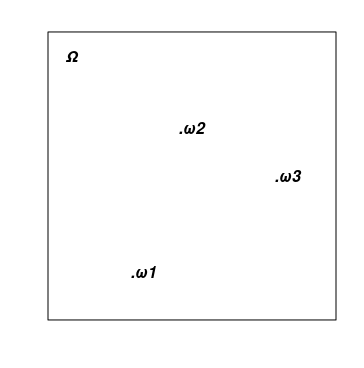2) A set of random events. Each such event is a set of elementary events (a subset of all Ω elementary events). The set of events includes an empty set ∅={} (an event that never happens) and the entire Ω set (an event that always happens). The combination (and intersection) of two events from the set should also belong to the set. In mathematics, such a collection of sets is usually called the algebra of sets. The coin example above has four events: {}=∅{H}, {T} and {H,T}=Ω. (Self-test question: Can an elementary event be considered an example of a random event?)

Random events are usually denoted as capital Latin letters: A, B, C, ... and depicted in images as shapes located inside Ω. The combination and intersection of events are denoted in different ways. Sometimes, an entry similar to addition and multiplication of ordinary numeric variables is used: АВ and А+В, while sometimes and ∪ signs are used: А∩В and АВ.

The image below shows Ω as a rectangle and two intersecting А and В events.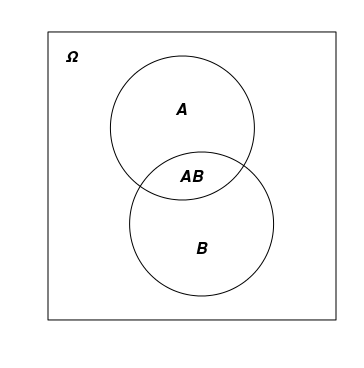3) Probability is a numerical function P=P(A) matching each A random event with a real number from 0 to 1. P(Ω)=1 and P(∅)=0. Besides, the additivity rule is fulfilled: if the A event is a combination of non-overlapping B and C events, then P(A)=P(B)+P(C). In addition to the "probability" term, the P() function applies the "probability distribution on Ω" (or simply "distribution on Ω"). Do not confuse this concept with the similar concept of the "distribution function of random variable". They are related to each other but still different. The former is a function matching a number to a set, while the latter one is an ordinary numeric function matching a number to a number.

It is not clear how to depict the probability distribution in an image, but intuitively it can be likened to the distribution of unit mass over the Ω volume. In this comparison, an event is part of the volume, while the probability is a share of the mass within this part of the volume.

All other probability theory concepts are derived from these. Let's highlight a very important concept of probabilistic dependence (independence) here. To do this, I am going to introduce the concept of the А event conditional probability subject to the B event execution, P(B)>0. It is denoted as P(A|B) and, by definition, P(A|B)=P(AB)/P(B) (as you may remember, AB means intersection of the A and B events). By definition, the A event is considered independent from the B event in the case when its conditional probability, during the B event occurrence, is equal to the probability: P(A|B)=P(A). If we use the conditional probability expression, the definition of independence can be rewritten as follows: P(A)P(B)=P(AB). If this equality is not satisfied, then the A event is said to depend on the B event.

Intuitively, independence means that knowing of the B event occurrence does not change the uncertainty associated with the A event. Conversely, dependence means that execution of the B event carries information about the A event. The precise expression of this intuitive understanding is given in Claude Shannon's information theory.

The elementary probability theory is usually highlighted separately. The difference between the elementary theory and the non-elementary one is that it considers sets of elementary events consisting of a finite number of elements. Consequently, the set of random events also turns out to be finite (self-test question: Why is this true?). This theory developed long before Kolmogorov axioms and did not really need it. The rest of the article will focus on this part of the theory. The non-elementary theory will be discussed in the next article.

### 3. Elementary theory

Since the number of elementary outcomes is finite, we can simply set the probability for events containing exactly one elementary event (unit set). We just need to make sure that the sum of all such probabilities is equal to one. The probability of any event will be equal to the sum of such probabilities. These initial probabilities are not necessarily equal, but we will start with such models, which are usually summarized under the name "combinatorial probability".

3.1. Combinatorial probability

Let Ω consist of exactly N elementary outcomes, then the probability of events containing them in the quantity of m is equal to m/N. Probability calculations here consist of counting the number of options. As a rule, combinatorial methods are used for this, hence the name. Here are some examples:

Example 1. Suppose that we have n various items. How many different ways are there to arrange them (line them up)? Answer: n!=1*2*3*....*(n-1)*n ways. Each way is called permutation, and each permutation is an elementary event. Thus, N=n! and the probability of an event consisting of m permutations is m/n! (m/N=m/n!).

Let's solve a simple problem: Define the probability that a given object will be in the first position after a random permutation. If the first place is occupied by a selected item, the remaining n-1 items can be placed on the remaining n-1 places in (n-1)! ways. Thus, m=(n-1)!, which means the desired probability is equal to m/N=m/n!=(n-1)!/n!=1/n.

Example 2. We have n various items. How many various sets of k (k<=n) items can we separate from them? Here are two possible options depending on whether we consider two sets, differing only in the item order, different. If yes, then the answer is n!/(n-k)! sets. If no, then k! times less: n!/((n-k)!*k!). A set considering the order is called assignment, a set with no consideration to order is called a combination. The assignment number creation, also known as the binomial coefficient equation, applies special symbols − there are two options shown in the following figure.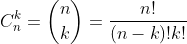Thus, if the order of elements within the set is not important, we can use combinations as a set of elementary events to solve the problem. If the order is important, then assignments should be used.

Example 3. Let's consider an important example leading to the so called hypergeometric distribution. Suppose that each of n items is marked either as "good", or as "bad". Let b, b⋜n of items be "bad", then the remaining n-b ones are "good". Select a set of k elements without taking their order into account (combination). What is the probability that our set contains exactly x "bad" items? The problem is solved by counting the number of matching combinations. The answer is rather cumbersome and it is better to write it down through the notations for the number of combinations as shown in the following figure where the desired probability is denoted via p and expressed via x, n, b and k.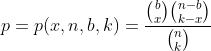This example is well suited for understanding the logic behind introducing the "random variable" concept (it will be considered in more detail in the next article). It may well turn out that in order to solve a specific problem related to calculating the probabilities of events, the knowledge of x, n, b and k is quite sufficient, while the full data on the entire initial set of events is redundant. Then it makes sense to simplify the original model by discarding unnecessary information. Let's proceed as follows:

• n, b and k are assumed to be fixed parameters.
• Instead of the Ω sample space, construct a new one Ωх={0, 1, ..., k} based on it. The new space is to consist of possible х values.
• Match each {х} event (consisting of one elementary event) with a probability specified by the hypergeometric distribution equation shown above.

The resulting object is called a "discrete random variable", which has a hypergeometric distribution for its possible values from Ωх.

3.2. Bernoulli scheme

This is another well-known model from the field of elementary probability theory. Its examples usually involve modeling the results of successive coin tosses, however I am going to construct the scheme in a more formal way.

Suppose that we have a positive integer n and a pair of non-negative real p and q, so that p+q=1. The Ω sample space consists of words having the exact length of n, in which only H and T letters are allowed (H − heads, T − tails). The probability for an event consisting of one elementary event is set by the equation pu({w})=p^nh*q^nt, where w is a word, while nh and nt, nh+nt=n stand for the number of H and T letters accordingly.

It is easy to see that, in contrast to the combinatorial probability, the initial probabilities are generally not equal to each other (they are all similar only if p=q=0.5).

Let's consider the n=2 as an example. In this case, Ω={HH, HT, TH, TT}. The number of elementary is equal to 4 here, while the number of random events is 16. (Self-test question: Derive the general form of the equations describing the dependence of the elementary event number and the number of all random events from n for the Bernoulli scheme).

Let's consider the "H"=comes first {HH, HT} event. Its probability is pq+p^2=p. The same is true for any position allowing us to talk about the p parameter as the "likelihood of getting tails on each toss". Now we check whether the А="H goes second"={HH, TH} event is independent from the В="H goes first"={HH, HT} event. Let's use the independence definition − the intersection of АВ={HH}, P(A)=p, P(B)=p and P(AB)=p^2. Since P(A)P(B)=p*p=p^2=P(AB), the events are independent.

The statements about the probability of the result of each toss and their independence turn out to be true for all n>2.

We could have specified the probability in some other way which could potentially lead either to the absence of the equal probability, or to the dependence of the toss results. The point here is that Bernoulli scheme is not the only valid model describing the sequence of events, and we should not confine ourselves to it.

Now let's calculate the probability of an event consisting of the words where H occurs exactly k times, or (less formally) the probability of tossing a head is equal to k times in n tosses. The answer to this question can be found via the so-called binomial distribution equation shown in the following figure. pb stands for the desired probability depending on k, n, p and q.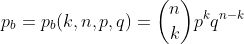Consider another example showing the relationship between the binomial distribution and the hypergeometric one considered above. It is important both in itself and in connection with its applications in mathematical statistics (Fisher's exact test). The question is quite complex and meaningful from a mathematical point of view. Let's highlight all the reasoning point by point.

• Based on the Bernoulli scheme's Ω sample space, construct the new one − Ω1 including only the words, in which H occurs exactly b times.
• Since any A event within Ω1 is also an event within Ω, the P(A) probability is defined for it. Based on that fact, let's introduce the P1 probability to Ω1 according to the P1(A)=P(A)/Р(Ω1) equation. The conditional probability equation P1(A)=P(A|О1) is, in fact, used here.
• Now consider the P1() probability of the event "the suffix of a word of k length contains exactly x number of H letters" from Ω1. It turns out that this probability is exactly set by the hypergeometric distribution equation provided above. Quite notably, the equation does not affect the p parameter.

### 4. Fundamentals of mathematical statistics

The difference between mathematical statistics and probability theory is usually described as a difference in the types of problems they solve. In probability theory, it is usually assumed that the probabilistic model is fully known and some conclusions should be drawn based on it. In mathematical statistics, knowledge about the model is incomplete, but there is additional information in the form of experimental data that helps refine the model. Thus, all questions discussed in the previous chapter are problems of the probability theory.

The definition of mathematical statistics I have just provided can be considered conventional. There is also another, more modern, approach to defining the mathematical statistics. It may be defined as a part of decision theory. In this case, the emphasis is made on constructing decision rules that are optimal in the sense of minimizing the average cost of an error. There is a very strong convergence with machine learning methods here. A significant difference from them lies in the fact that in mathematical statistics, the type of the applied mathematical model is determined quite clearly (for example, within the accuracy of an unknown parameter). In machine learning, uncertainty usually extends to the model type as well.

Now let's consider sample problems of mathematical statistics in its conventional sense.

### 5. Examples of applying mathematical statistics within the framework of the elementary theory

There are two types of problems − estimating a parameter and checking hypotheses.

Let's start from the parameter point estimation. It assumes the presence of any numerical (non-random, deterministic) variables in the probabilistic model. Their exact numerical value is unknown, but we can calculate their approximate value using data obtained as a result of a random experiment.

5.1. Parameter point estimation

The most general approach here is to use the maximum likelihood estimation method. If some ω elementary event has been realized as a result of a random experiment, the likelihood function is the probability of the {ω} event (consisting of this elementary event only). It is called a function insofar as it depends on the model parameter value. The maximum likelihood estimation (MLE) is the parameter value, at which this function reaches its maximum.

There may be multiple various parameter estimations out there in addition to MLE but, as proved in mathematical statistics, MLE is the best one in terms of accuracy. I will leave the explanation of what is meant by the word "precision" here until the next article devoted to random variables. In any case, we should keep in mind that the statistical estimation is almost always different from the true value of the parameter. Therefore, it is very important to distinguish between them. For example, the probability of an event in the Bernoulli scheme and its estimation in the form of a frequency.

Let's move on to calculating the MLE using examples.

Example 1. Estimating the b parameter in the hypergeometric distribution. There is a batch of workpieces n=1000 pcs. After checking k=20 of them, a defective workpiece has been detected: x=1. Estimate the number of defective workpieces in the entire batch.

Below is hyperg_be.py script written in Python, which solves this problem by enumerating all possible options for b. The answer is be estimation, in which the likelihood value, determined by the hypergeometric distribution equation, is maximum.

```from scipy.stats import hypergeom

n = 1000
k = 20
x = 1
lhx = 0.0
be = 0
for b in range(x, n - k + x):
lh = hypergeom.pmf(x, n, b, k)
if lh > lhx:
be = b
lhx = lh

print("be =",be)

```

Answer: be = 50, which is quite expected (every 20 th workpiece).

Example 2. Estimating the n parameter in the hypergeometric distribution. It is required to estimate the amount of fish in the water body. To do this, b=50 fishes were caught with a net, marked and released back. After that, k=55 fishes were caught, of which x=3 turned out to be marked.

Below is hyperg_ne.py script written in Python, which solves this problem by enumerating possible options for n. The answer is ne estimation with the highest probability value. A little nuance is that the possible values for n theoretically range from 50+(55-3)=102 to infinity. This could lead to an endless enumeration loop. But it turns out that the likelihood function grows till some n value. Then it starts decreasing and tends to zero. Accordingly, the answer is the first ne value, at which the likelihood function value is greater than its value by ne+1.

```from scipy.stats import hypergeom

b = 50
k = 55
x = 3
lh0 = 0.0
ne = b + k - x - 1
while True:
lh = hypergeom.pmf(x, ne+1, b, k)
if lh < lh0:
break
else:
lh0 = lh
ne += 1

print("ne =",ne)

```

Answer: ne = 916, which is also quite expected (ne/b is roughly equal to k/x, whence it turns out that ne is roughly equal to b*k/x).

All examples below are related to the Bernoulli scheme or its modifications. The conventional trading interpretation of this model involves matching it with a discretized version of an asset price. For example, a renko or point and figure chart may be used to obtain such a representation.

Let's adhere to this conventional method. Instead of H and T letters, we are going to have a look at sequences of 1 and -1 numbers, which obviously correspond to discrete price steps (up and down, respectively). Generally, the price step can be defined as reaching a new level on a price grid. These level grids are usually defined so that either the difference or the relationship between each level and its lower neighbor remains the same. I will use the second method. The disadvantage of this method is the impossibility of its application for assets with negative prices, while the advantage is the absence of the need to select a step size for each asset individually. Also, there is some arbitrariness in the choice of a zero level. Its part will be played by the very first price of those subject to discretization.

Below is a simple script that discretizes the price with a given percentage step at a given interval. The Discr.mqh file contains only the setmv() function, in which the price discretization actually occurs. For simplicity, only open prices of minute bars are taken as starting prices.

```// Constructing the array of discrete mv[] movements
// at a specified time interval and with a specified percentage step
void setmv(int& mv[], datetime t1, datetime t2, double dpr)
{
int ND = 1000;
ArrayResize(mv, 0, ND);
// Get price history
double price[];
int nprice = CopyOpen(Symbol(), PERIOD_M1, t1, t2, price);
if(nprice < 2)
{
Print("not enough price history");
return;
}
// Construct mv[]
int lvl = 0, dlvl, nmv = 0, dmv;
double lp0 = log(price), lstep = log(1 + 0.01 * dpr);
for(int i = 1; i < nprice; ++i)
{
dlvl = (int)((log(price[i]) - lp0) / lstep - lvl);
if(dlvl == 0) continue;
lvl += dlvl;
dmv = 1;
if(dlvl < 0)
{
dmv = -1;
dlvl = -dlvl;
}
ArrayResize(mv, nmv + dlvl, ND);
for(int j = 0; j < dlvl; ++j) mv[nmv + j] = dmv;
nmv += dlvl;
}
}

```

The discret_prices.mq5 script displays the result as a sequence of 1 and -1, as well as a chart of a discrete analogue of the starting price.

```#include <Discr.mqh>
#include <Graphics\Graphic.mqh>
#property script_show_inputs
//+------------------------------------------------------------------+
input datetime tstart = D'2020.05.20 00:00';  // start of the considered time interval
input datetime tstop = D'2020.06.20 00:00';   // end of the considered time interval
input double   dprcnt = 0.5;                  // price discretization step in %
//+------------------------------------------------------------------+
void OnStart()
{
int mv[], nmv;
setmv(mv, tstart, tstop, dprcnt);
nmv = ArraySize(mv);
if(nmv < 1)
{
Print("not enough moves");
return;
}
// Display mv[] as a sequence of 1 and -1
string res = (string)mv;
for(int i = 1; i < nmv; ++i) res += ", " + (string)mv[i];
Print(res);
// Display the mv[] cumulative sum chart
ChartSetInteger(0, CHART_SHOW, false);
CGraphic graphic;
graphic.Create(0, "G", 0, 0, 0, 750, 350);
double x[], y[];
ArrayResize(x, nmv + 1);
ArrayResize(y, nmv + 1);
x = y = 0.0;
for(int i = 1; i <= nmv; ++i)
{
x[i] = i;
y[i] = y[i-1] + mv[i-1];
}
ArrayPrint(x);
ArrayPrint(y);
graphic.CurvePlotAll();
graphic.Update();
Sleep(30000);
ChartSetInteger(0, CHART_SHOW, true);
graphic.Destroy();
}

```

In all examples below, I will use the results of EURUSD price discretization with the increment of 0.5% from May 20 to June 20, 2020. The result is the following sequence of discrete steps: 1, -1, 1, 1, -1, 1, 1, 1, -1, 1, 1, 1, -1, 1, 1, 1, -1, -1, 1, 1, 1, -1, -1, -1, 1, -1, -1. The charts of the original price and its discrete analogue are displayed below for more clarity.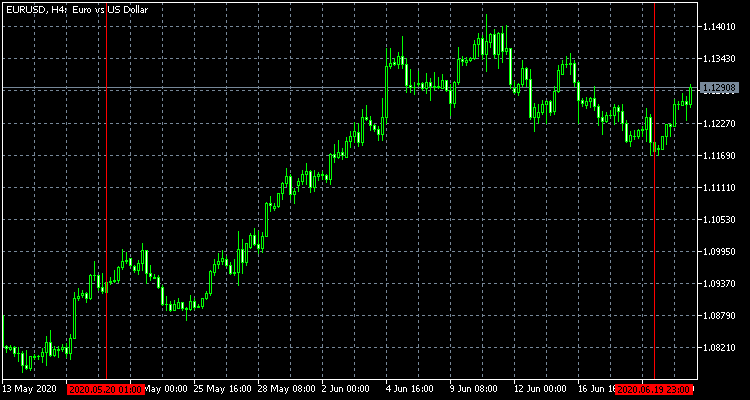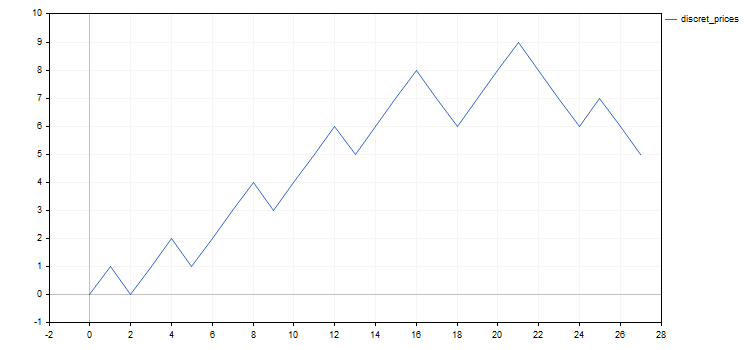In addition to studying the behavior of the discretized price, there may be other ways to apply the Bernoulli scheme or its modifications. Let's point out two of them related to trading.

1. Results of trading, during which deals are made with fixed stop loss, take profit and volume levels. All profits in a series of trades are approximately equal to each other, and the same is true for losses. Therefore, the probability of a profit is a sufficient parameter to describe the trading result. For example, we can raise a question of whether the probability of making a profit is high enough in the existing sequence of deals.
2. Selecting assets for investment. Suppose that we have a large selection of investment methods at the start of the period. Any of them, with some probability, can eventually lead to a complete loss of invested funds. If there is some way of selecting assets, then the question can be raised about the difference in the probability of bankruptcy among the selected assets in comparison with that among the rejected ones.

Example 3. Estimating the p parameter in the Bernoulli scheme. This is the rare case where a problem can be solved on paper. The likelihood function looks as follows: p^nup*(1-p)^ndn. Take the derivative with respect to p and equate it to zero to get the expected value for estimating р as a frequency: pe=nup/(nup+ndn)=nup/nmv. As is usually the case, the search is performed not for the likelihood function maximum but its logarithm, which is much easier. The answer is the same since the logarithm is a strictly increasing function, and the maximum is reached with the same parameter value. Below is the p_model.mq5 script calculating this estimation.

```#include <Discr.mqh>
#property script_show_inputs
//+------------------------------------------------------------------+
input datetime tstart = D'2020.05.20 00:00';  // start of the considered time interval
input datetime tstop = D'2020.06.20 00:00';   // end of the considered time interval
input double   dprcnt = 0.5;                  // price discretization step in %
//+------------------------------------------------------------------+
void OnStart()
{
int mv[];
setmv(mv, tstart, tstop, dprcnt);
if(ArraySize(mv) < 1)
{
Print("not enough moves");
return;
}
double pe = calcpe(mv);
Print("pe=", pe);
}
//+------------------------------------------------------------------+
// Calculate the probability estimation
double calcpe(int& mv[])
{
int nmv = ArraySize(mv);
if(nmv < 1) return 0.0;
int nup = 0;
for(int i = 0; i < nmv; ++i) if(mv[i] > 0) ++nup;
return ((double)nup) / nmv;
}
```

Example 4. Estimating the parameter in the modified Bernoulli scheme. As I wrote above, Bernoulli scheme can very well be changed if required for our modeling purposes. Let's consider a possible modification option.

Perhaps, the easiest option is to divide the sequence of movements into two smaller ones going one after another and corresponding to the Bernoulli scheme with their parameters: n1, p1, n2=n-n1 and p2, where n is the length of the cumulative sequence.
Thus, three parameters need to be estimated n1, p1 and p2. Maximizing the log-likelihood function relative to p1 and p2 allows us to express them analytically via n1. The estimation of n1 should be found by a simple search substituting expressions for p1 and p2 to the log-likelihood equation.
Below is the script for p1p2_model.mq5 calculating parameter estimations.

```#include <Discr.mqh>
#property script_show_inputs
//+------------------------------------------------------------------+
input datetime tstart = D'2020.05.20 00:00';  // start of the considered time interval
input datetime tstop = D'2020.06.20 00:00';   // end of the considered time interval
input double   dprcnt = 0.5;                  // price discretization step in %
//+------------------------------------------------------------------+
void OnStart()
{
int mv[];
setmv(mv, tstart, tstop, dprcnt);
if(ArraySize(mv) < 2)
{
Print("not enough moves");
return;
}
double p1e, p2e;
int n1e;
calc_n1e_p1e_p2e(mv, n1e, p1e, p2e);
Print("n1e=", n1e, " p1e=", p1e, " p2e=", p2e);
}
//+------------------------------------------------------------------+
// Calculate the probability estimation
void calc_n1e_p1e_p2e(int& mv[], int& n1e, double& p1e, double& p2e)
{
n1e = 0;
p1e = p2e = 0.0;
int nmv = ArraySize(mv);
if(nmv < 2) return;
n1e = 1;
double llhx = llhx_n1(mv, 1, p1e, p2e), llh, p1, p2;
for(int n1 = 2; n1 < nmv; ++n1)
{
llh = llhx_n1(mv, n1, p1, p2);
if(llh > llhx)
{
llhx = llh;
n1e = n1;
p1e = p1;
p2e = p2;
}
}
}
//+------------------------------------------------------------------+
// log-likelihood function maximum depending on n1
double llhx_n1(int& mv[], int n1, double& p1, double& p2)
{
p1 = p2 = 0.0;
int nmv = ArraySize(mv);
if(nmv < 2 || n1 < 1 || n1 >= nmv) return 0.0;
int nu1 = 0, nu2 = 0;
for(int i = 0; i < n1; ++i) if(mv[i] > 0) ++nu1;
for(int i = n1; i < nmv; ++i) if(mv[i] > 0) ++nu2;
double l = 0.0;
if(nu1 > 0)
{
p1 = ((double)nu1) / n1;
l += nu1 * log(p1);
}
if(nu1 < n1) l += (n1 - nu1) * log(((double)(n1 - nu1)) / n1);
if(nu2 > 0)
{
p2 = ((double)nu2) / (nmv - n1);
l += nu2 * log(p2);
}
if(nu2 < nmv - n1) l += (nmv - n1 - nu2) * log(((double)(nmv - n1 - nu2)) / (nmv - n1));
return l;
}
```

Answer: n1e = 21; p1e = 0.71; p2e = 0.17 (numbers rounded). It seems quite obvious our model "detected" the price direction change (or correction) at the end of its segment. This suggests that the transition to a more complex model in this case was not in vain.

Example 5. In the previous example, the probability of the upward movement depended on its number (in time). Let's consider another modification of the Bernoulli scheme, in which this probability depends on the previous movement itself. Let's construct a model which is an example of the simplest Markov chain with two states.

Suppose that the movements are numbered from 0 to n. For zero movement, the probability of upward movement is simply set equal 0.5 since there is no previous movement for it. For other positions, the probability of an upward movement is equal to p1, if it was preceded by an upward movement, and p2, if it was preceded by a downward movement. The probabilities of downward movements in these two cases are equal to q1=1-p1 and q2=1-p2 accordingly. For example, the probability of an event having one elementary event "down-up-up-down" is 0.5*p2*p1*q1.

We have obtained a model with two parameters that can be estimated using the MLE. Here all calculations can be done on paper again especially if we maximize the log-likelihood function. As in the case of the Bernoulli scheme, the answer boils down to frequencies. The number of times the downward movement occurs after the upward one is set as nud (u up, d down). In a similar way, introduce nuu, ndd and ndu. Estimations for p1 and p2 are denoted via p1e and p2e. Then these estimations are found using the equations p1e=nuu/(nuu+nud) and p2e=ndu/(ndu+ndd).

Below is the markov_model.mq5 script calculating these estimations.

```#include <Discr.mqh>
#property script_show_inputs
//+------------------------------------------------------------------+
input datetime tstart = D'2020.05.20 00:00';  // start of the considered time interval
input datetime tstop = D'2020.06.20 00:00';   // end of the considered time interval
input double   dprcnt = 0.5;                  // price discretization step in %
//+------------------------------------------------------------------+
void OnStart()
{
int mv[];
setmv(mv, tstart, tstop, dprcnt);
if(ArraySize(mv) < 2)
{
Print("not enough moves");
return;
}
double p1e, p2e;
calcpes(mv, p1e, p2e);
Print("p1e=", p1e, " p2e=", p2e);
}
//+------------------------------------------------------------------+
// Calculate probability estimations
void calcpes(int& mv[], double& p1e, double& p2e)
{
p1e = p2e = 0;
int nmv = ArraySize(mv);
if(nmv < 2) return;
int nuu = 0, nud = 0, ndu = 0, ndd = 0, nu, nd;
for(int i = 0; i < nmv - 1; ++i)
if(mv[i] > 0)
{
if(mv[i + 1] > 0) ++nuu;
else ++nud;
}
else
{
if(mv[i + 1] > 0) ++ndu;
else ++ndd;
}
nu = nuu + nud;
nd = ndu + ndd;
if(nu > 0) p1e = ((double)nuu) / nu;
if(nd > 0) p2e = ((double)ndu) / nd;
}
```

Answer:  p1e = 0.56; p2e = 0.60 (numbers rounded). Both probabilities being almost equal to each other indicates that there is no dependence between adjacent movements. Both probabilities being greater than 0.5 indicates the upward trend. Both probability estimations being close to the probability estimation for a simpler model (the simple Bernoulli scheme from the third example) indicate that the transition to this complicated model has turned out to be unnecessary in this particular case.

5.2. Testing statistical hypotheses

Different probability distributions can be set on the same sample space. A hypothesis is a statement that specifies the type of distribution. There should be several hypotheses (at least two) since the solution lies in the preference of one hypothesis over the others. A hypothesis is called simple if it uniquely determines the type of distribution.

Let's provide examples for the Bernoulli scheme: the hypothesis of the p parameter equality to a certain number − a simple hypothesis (only one possible parameter value unambiguously defining the distribution), while the hypothesis that the parameter exceeds the specified number is a complex hypothesis (infinite amount of possible parameter values).

Next, we will deal with the selection of one of the two hypotheses. One of them is called null, and the other is alternative. The hypothesis is chosen using a statistical criterion. Basically, this is just a function with 0 and 1 values within the sample space, where 0 means accepting the null hypothesis, while 1 indicates accepting the alternative one.

Thus, we can either accept the null hypothesis or reject it. Accordingly, the alternative hypothesis is either rejected or accepted. Each of these decisions can be either right or wrong. Thus, there are four possible outcomes of accepting the hypothesis, of which two are correct and two are false.

1. Type 1 error − erroneous rejection of the null hypothesis.
2. Type 2 error − erroneous acceptance of the null hypothesis.

The probabilities of type 1 and 2 errors are denoted via a1 and a2. Naturally, it would be good to lessen these probabilities as much as possible. But unfortunately, this is impossible. Moreover, by decreasing the probability of errors of a certain type, we quite expectedly face the increase of the probability of errors of another type. This is why a certain compromise between these errors is usually made.

1-a1 is called the test significance, while 1-a2 is called its power. The test power can be difficult to calculate, especially for a complex hypothesis. Therefore, only the test significance is usually used.

A criterion is generally based on a numerical function (also defined based on a sample space) called test statistic. Its multiple values are divided into two areas. One of the areas corresponds to the acceptance of the null hypothesis, while the other corresponds to its rejection. The area, in which the null hypothesis is rejected, is called critical.

Below is an important note about the "minus first" hypothesis. This term means that we should make sure in advance that one of our two hypotheses is fulfilled. Roughly speaking, if we separate Chihuahuas from dogs of other breeds by weight, then we must be sure that we always have a dog on the scales (not an elephant, mouse or cat). Otherwise, our test is meaningless. This principle is most often violated when statistical criteria used in econometrics are applied inaccurately.

Below are the examples of testing hypotheses for the Bernoulli scheme.

Example 1. Testing the null hypothesis p=p0 in the Bernoulli scheme. This hypothesis is simple. The alternative hypothesis can be one of the following two options (it is complex in both cases). kup (number of upward movements in the sequence) is used as the statistics criterion in both cases, although the critical area is defined differently.

1. p>p0, the critical area is to the right kup⋝kr
2. p<p0, the critical area is to the left kup⋜kl

Specific values for kr and kl are found using the condition that the probability of the statistics falling into the critical area does not exceed the selected probability of type 1 error. As I have already mentioned, all these probabilities are calculated under the condition that the null hypothesis is fulfilled (by the Bernoulli distribution with the parameter p=p0).

Let's check this hypothesis for p0=0.5 on the same discretized sequence of prices. The value for p0 is great in that it fits the "random walk" ("fair coin") hypothesis.

Below is the script p0_hypothesis.mq5 testing this hypothesis.

```#include <Math\Stat\Binomial.mqh>
#property script_show_inputs
//+------------------------------------------------------------------+
input int    nm = 27;          // total number of movements in the series
input int    kup = 16;         // number of upward movements
input double p0 = 0.5;         // checked probability value
input double alpha1 = 0.05;    // type 1 error probability
//+------------------------------------------------------------------+
void OnStart()
{
int kl, kr, er;
kr=(int)MathQuantileBinomial(1.0-alpha1,nm,p0,er);
kl=(int)MathQuantileBinomial(alpha1,nm,p0,er);
Print("kl=", kl, " kup=", kup, " kr=", kr);
Print("kup<=kl = ", kup<=kl);
Print("kup>=kr = ", kup>=kr);
}
```

Response:

• kl=9; kup=16; kr=18
• kup<=kl = false
• kup>=kr = false

The result indicates that the null hypothesis cannot be rejected at this level of significance in favor of any of the alternatives.

Example 2. Suppose that we have two sequences with the lengths of n1 and n2 taken from two Bernoulli schemes having the p1 and p2 parameters. The null hypothesis here lies in the statement that the p1=p2 equality is fulfilled, while the possible alternatives are different types of their inequality, of which there are also two here. k2 is used as the statistics criterion in all these cases − number of upward movements in the second sequence out of two ones, although the critical area is defined differently.

• p2>p1, the critical area is to the right k2⋝kr
• p2<p1, the critical area is to the left k2⋜kl

The significant difference from the previous example is that here we are talking not about statements related to accurate p1 and p2 values, but only about their ratio. The critical regions are defined in the same way as in the previous example, but the distribution of statistics under the null hypothesis is hypergeometric, not binomial. In mathematical statistics, this criterion is known as Fisher's exact test.

Below is the p1p2_hypothesis.py script in Python solving this problem for the discretized price split in two. I use the split that was obtained in the fourth example from the previous paragraph.

```from scipy.stats import hypergeom

n1 = 21; n2 = 6
k1 = 15; k2 = 1
alpha1 = 0.05

kl = int(hypergeom.ppf(alpha1, n1 + n2, k1 + k2, n2))
kr = int(hypergeom.ppf(1.0 - alpha1, n1 + n2, k1 + k2, n2))

print("kl =", kl, " k2 =", k2, " kr =", kr)
print("k2<=kl =", k2<=kl)
print("k2>=kr =", k2>=kr)
```

Response:

• kl = 2;  k2 = 2;  kr = 6
• k2<=kl = True
• k2>=kr = False
The result indicates that the null hypothesis is rejected at this level of significance in favor of the first alternative. This is an additional confirmation that our price section can be split into two sections with the price moving in different directions.

### 6. Conclusion

The article has not covered such important areas of mathematical statistics as descriptive statistics, interval parameter estimation, asymptotic estimates and the Bayesian approach in statistics. The reason is that I need to clarify the concept of a random variable for their meaningful consideration. I have decided to do that in the next article to make the current one compact enough.

### 7. Attached files

#
Name
Type Description
1 hyperg_be.py   Python script  Calculating the b hypergeometric distribution parameter estimation. Example 5.1.1
2 hyperg_ne.py   Python script   Calculating the n hypergeometric distribution parameter estimation. Example 5.1.2
3  Discr.mqh  MQL5 header file  The file with the setmv() function used in all MQL5 examples below for the price discretization
4  discret_prices.mq5  MQL5 script  The script performing the price discretization and displaying the result as a sequence of 1 and -1 and as a chart
5  p_model.mq5  MQL5 script  Calculating the p parameter estimation in the Bernoulli scheme. Example 5.1.3
6  p1p2_model.mq5  MQL5 script  Calculating n1, p1 and p2 parameter estimations in the model consisting of two Bernoulli schemes. Example 5.1.4
7  markov_model.mq5  MQL5 script  Calculating p1 and p2 parameter estimations of the Markov chain. Example 5.1.5
8  p0_hypothesis.mq5  MQL5 script
Checking the hypothesis of the Bernoulli scheme parameter being equal to a specified number. Example 5.2.1
9  p1p2_hypothesis.py  Python script  Fisher's exact test. Example 5.2.2

Translated from Russian by MetaQuotes Software Corp.
Original article: https://www.mql5.com/ru/articles/8038

Attached files |
hyperg_be.py (0.22 KB)
hyperg_ne.py (0.24 KB)
Discr.mqh (1.71 KB)
discret_prices.mq5 (2.67 KB)
p_model.mq5 (1.86 KB)
p1p2_model.mq5 (3.95 KB)
markov_model.mq5 (2.51 KB)
p0_hypothesis.mq5 (1.83 KB)
p1p2_hypothesis.py (0.45 KB)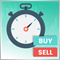Quick Manual Trading Toolkit: Working with open positions and pending orders

In this article, we will expand the capabilities of the toolkit: we will add the ability to close trade positions upon specific conditions and will create tables for controlling market and pending orders, with the ability to edit these orders.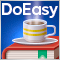Timeseries in DoEasy library (part 46): Multi-period multi-symbol indicator buffers

In this article, I am going to improve the classes of indicator buffer objects to work in the multi-symbol mode. This will pave the way for creating multi-symbol multi-period indicators in custom programs. I will add the missing functionality to the calculated buffer objects allowing us to create multi-symbol multi-period standard indicators.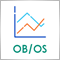On Methods to Detect Overbought/Oversold Zones. Part I

Overbought/oversold zones characterize a certain state of the market, differentiating through weaker changes in the prices of securities. This adverse change in the synamics is pronounced most at the final stage in the development of trends of any scales. Since the profit value in trading depends directly on the capability of covering as large trend amplitude as possible, the accuracy of detecting such zones is a key task in trading with any securities whatsoever.Timeseries in DoEasy library (part 47): Multi-period multi-symbol standard indicators

In this article, I will start developing the methods of working with standard indicators, which will ultimately allow creating multi-symbol multi-period standard indicators based on library classes. Besides, I will add the "Skipped bars" event to the timeseries classes and eliminate excessive load from the main program code by moving the library preparation functions to CEngine class.